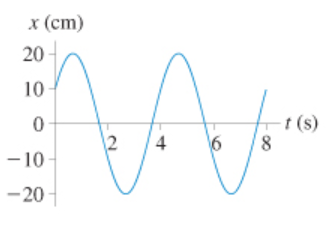# Problem: Part AWhat is the amplitude of the oscillation shown in the figure? (Figure 1)Express your answer to three significant figures and include the appropriate units.Part BWhat is the frequency of this oscillation?Express your answer to two significant figures and include the appropriate units.Part CWhat is the phase constant?Express your answer to two significant figures.

###### FREE Expert Solution

The amplitude:

$\overline{){\mathbf{A}}{\mathbf{=}}{{\mathbf{x}}}_{\mathbf{m}\mathbf{a}\mathbf{x}}}$

Frequency:

$\overline{){\mathbf{F}}{\mathbf{=}}\frac{\mathbf{1}}{\mathbf{T}}}$

The general wave equation of a sinusoidal wave:

$\overline{){\mathbf{x}}{\mathbf{=}}{\mathbf{A}}{\mathbf{c}}{\mathbf{o}}{\mathbf{s}}{\mathbf{\left(}}{\mathbf{\omega }}{\mathbf{t}}{\mathbf{+}}{\mathbf{\varphi }}{\mathbf{\right)}}}$

Part A

From the graph, the maximum value of x is 20 cm

88% (176 ratings)###### Problem DetailsPart A

What is the amplitude of the oscillation shown in the figure? (Figure 1)

Express your answer to three significant figures and include the appropriate units.

Part B

What is the frequency of this oscillation?

Express your answer to two significant figures and include the appropriate units.

Part C

What is the phase constant?